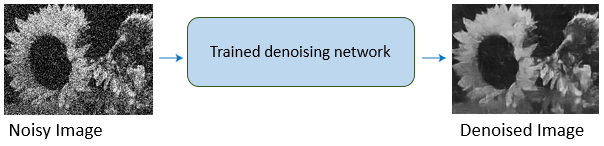Main Content

# Deep Learning for Image Processing

Perform image processing tasks, such as removing image noise and creating high-resolution images from low-resolutions images, using convolutional neural networks (requires Deep Learning Toolbox™)

Deep learning uses neural networks to learn useful representations of features directly from data. For example, you can use a pretrained neural network to identify and remove artifacts like noise from images.## Functions

expand all

 `augmentedImageDatastore` Transform batches to augment image data `blockedImageDatastore` Datastore for use with blocks from `blockedImage` objects `denoisingImageDatastore` Denoising image datastore `imageDatastore` Datastore for image data `randomPatchExtractionDatastore` Datastore for extracting random 2-D or 3-D random patches from images or pixel label images `transform` Transform datastore `combine` Combine data from multiple datastores
 `jitterColorHSV` Randomly alter color of pixels `randomWindow2d` Randomly select rectangular region in image `randomCropWindow3d` Create randomized cuboidal cropping window `centerCropWindow2d` Create rectangular center cropping window `centerCropWindow3d` Create cuboidal center cropping window `Rectangle` Spatial extents of 2-D rectangular region `Cuboid` Spatial extents of 3-D cuboidal region `randomAffine2d` Create randomized 2-D affine transformation `randomAffine3d` Create randomized 3-D affine transformation `affineOutputView` Create output view for warping images `imerase` Remove image pixels within rectangular region of interest
 `resize2dLayer` 2-D resize layer `resize3dLayer` 3-D resize layer `dlresize` Resize spatial dimensions of `dlarray` object `DepthToSpace2DLayer` Depth to space layer `SpaceToDepthLayer` Space to depth layer `depthToSpace` Rearrange `dlarray` data from depth dimension into spatial blocks `spaceToDepth` Rearrange spatial blocks of `dlarray` data along depth dimension
 `encoderDecoderNetwork` Create encoder-decoder network `blockedNetwork` Create network with repeating block structure `pretrainedEncoderNetwork` Create encoder network from pretrained network `cycleGANGenerator` Create CycleGAN generator network for image-to-image translation `patchGANDiscriminator` Create PatchGAN discriminator network `pix2pixHDGlobalGenerator` Create pix2pixHD global generator network `addPix2PixHDLocalEnhancer` Add local enhancer network to pix2pixHD generator network `unitGenerator` Create unsupervised image-to-image translation (UNIT) generator network `unitPredict` Perform inference using unsupervised image-to-image translation (UNIT) network
 `denoiseImage` Denoise image using deep neural network `denoisingNetwork` Get image denoising network `dnCNNLayers` Get denoising convolutional neural network layers

## Topics

### Preprocess Images for Deep Learning

Datastores for Deep Learning (Deep Learning Toolbox)

Learn how to use datastores in deep learning applications.

Augment Images for Deep Learning Workflows Using Image Processing Toolbox (Deep Learning Toolbox)

This example shows how MATLAB® and Image Processing Toolbox™ can perform common kinds of image augmentation as part of deep learning workflows.

Preprocess Images for Deep Learning (Deep Learning Toolbox)

Learn how to resize images for training, prediction, and classification, and how to preprocess images using data augmentation, transformations, and specialized datastores.

Preprocess Volumes for Deep Learning (Deep Learning Toolbox)

Read and preprocess volumetric image and label data for 3-D deep learning.

### Create Neural Networks for Image Processing Applications

Create Modular Neural Networks

You can create and customize deep learning networks that follow a modular pattern with repeating groups of layers, such as U-Net and cycleGAN.

Get Started with GANs for Image-to-Image Translation

GAN networks can transfer the styles and characteristics from one set of images to the scene content of other images.

### Denoise Images Using Deep Learning

Train and Apply Denoising Neural Networks

Use a pretrained neural network to remove Gaussian noise from a grayscale image, or train your own network using predefined layers.

Remove Noise from Color Image Using Pretrained Neural Network

This example shows how to remove Gaussian noise from an RGB image by using a pretrained denoising neural network on each color channel independently.

Prepare Datastore for Image-to-Image Regression (Deep Learning Toolbox)

This example shows how to prepare a datastore for training an image-to-image regression network using the `transform` and `combine` functions of `ImageDatastore`.

### Deep Learning in MATLAB

Deep Learning in MATLAB (Deep Learning Toolbox)

Discover deep learning capabilities in MATLAB® using convolutional neural networks for classification and regression, including pretrained networks and transfer learning, and training on GPUs, CPUs, clusters, and clouds.

Pretrained Deep Neural Networks (Deep Learning Toolbox)

Learn how to download and use pretrained convolutional neural networks for classification, transfer learning and feature extraction.

Semantic Segmentation Using Deep Learning (Computer Vision Toolbox)

This example shows how to train a semantic segmentation network using deep learning.

List of Deep Learning Layers (Deep Learning Toolbox)

Discover all the deep learning layers in MATLAB.

Download ebook